# 【数据结构】二叉树的全部详解，没有比这一篇更详细的了。_Layman光～的博客

### 二叉树

• 1.树的概念及结构
• 1.1树的概念
• 1.2树的相关概念
• 1.3树的表示
• 2.二叉树概念及结构
• 2.1概念
• 2.2特殊的二叉树
• 2.3二叉树的性质
• 2.4二叉树的存储结构
• 3.二叉树的顺序结构及实现
• 3.1二叉树的顺序结构
• 3.2堆的概念及结构
• 3.3堆的实现
• 3.3.1堆向下调整算法
• 3.3.2堆的创建
• 3.3.3建堆时间复杂度
• 3.3.4堆的插入
• 3.3.5堆的删除
• 3.3.6堆的代码实现
• 3.4堆的应用
• 3.4.1堆排序
• 3.4.2TOP-K问题
• 4.二叉树链式结构的实现
• 4.1前置说明
• 4.2二叉树的遍历
• 4.3二叉树的实现

# 1.树的概念及结构

## 1.1树的概念

1、有一个特殊的结点，称为根结点，根节点没有前驱结点。
2、除根节点外，其余结点被分成M(M>0)个互不相交的集合T1、T2、……、Tm，其中每一个集合Ti(1<= i<= m)又是一棵结构与树类似的子树。每棵子树的根结点有且只有一个前驱，可以有0个或多个后继。
3、因此，树是递归定义的。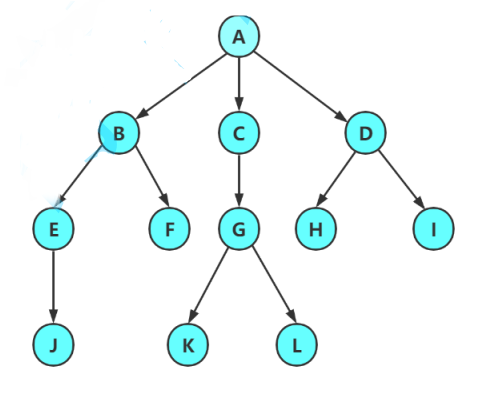## 1.2树的相关概念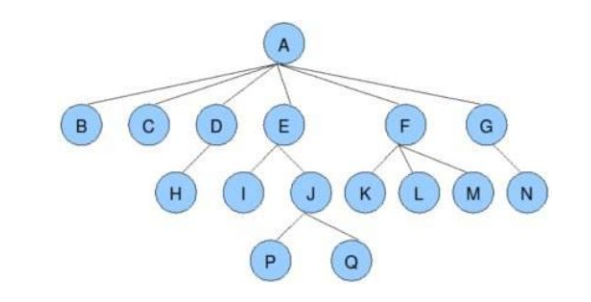## 1.3树的表示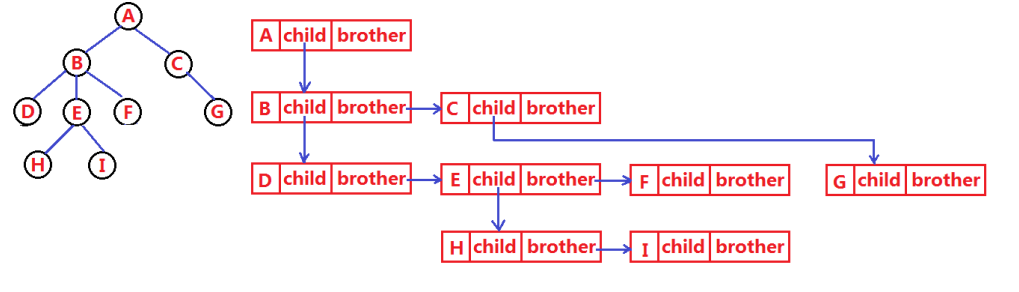``````typedef int DataType;
struct Node
{
struct Node* _firstChild1; // 第一个孩子结点
struct Node* _pNextBrother; // 指向其下一个兄弟结点
DataType _data; // 结点中的数据域
};
``````

# 2.二叉树概念及结构

## 2.1概念

1.或者为空
2. 由一个根节点加上两棵别称为左子树和右子树的二叉树组成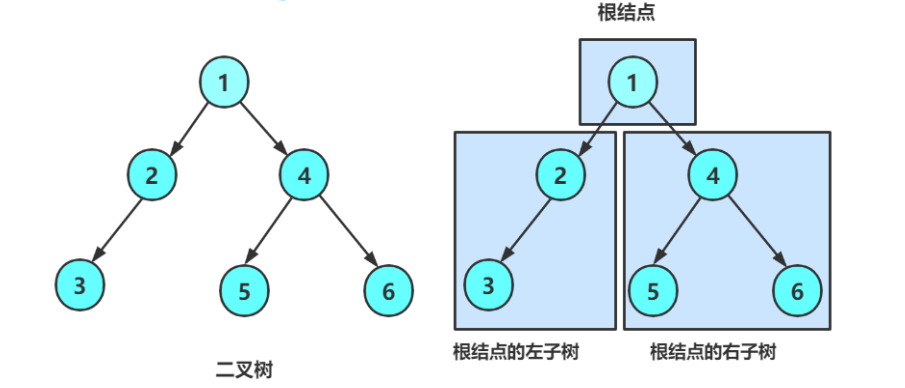1 .二叉树不存在度大于2的结点
2. 二叉树的子树有左右之分，次序不能颠倒，因此二叉树是有序树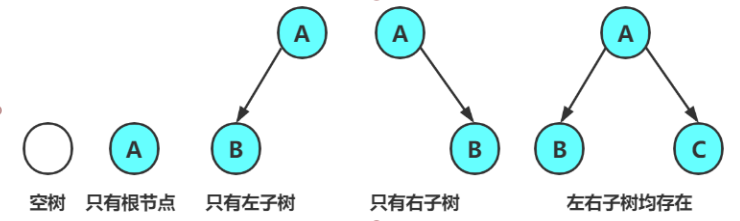## 2.2特殊的二叉树

1. 满二叉树：一个二叉树，如果每一个层的结点数都达到最大值，则这个二叉树就是满二叉树。也就是说，如果一个二叉树的层数为K，且结点总数是 ，则它就是满二叉树。
2. 完全二叉树：完全二叉树是效率很高的数据结构，完全二叉树是由满二叉树而引出来的。对于深度为K的，有n个结点的二叉树，当且仅当其每一个结点都与深度为K的满二叉树中编号从1至n的结点一一对应时称之为完全二叉树。 要注意的是满二叉树是一种特殊的完全二叉树。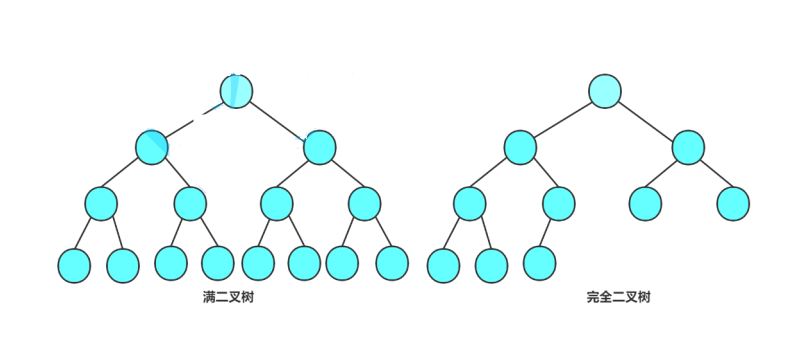## 2.3二叉树的性质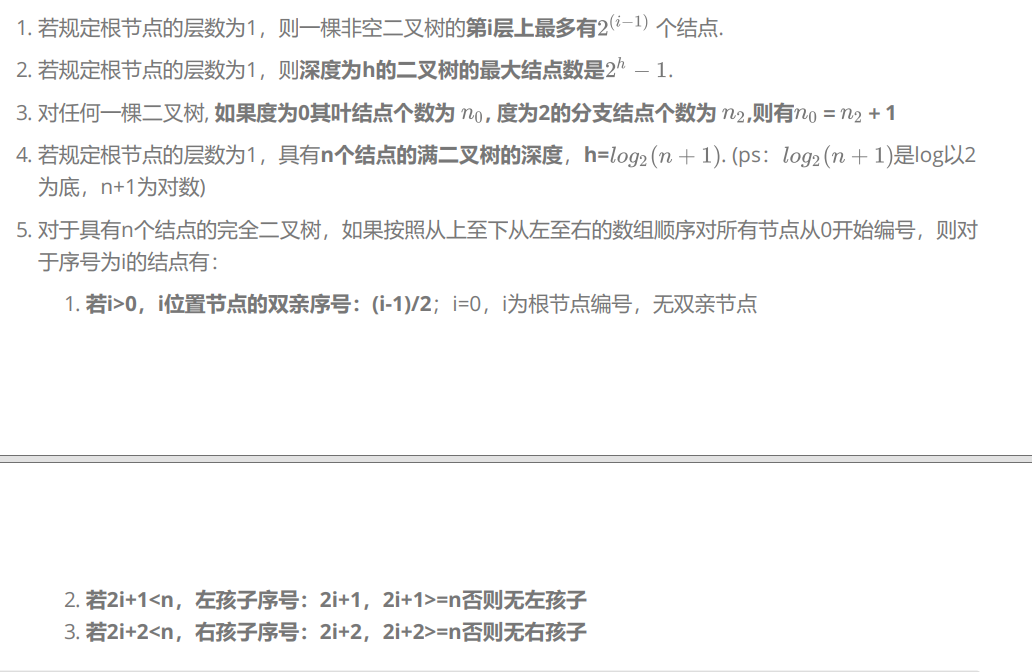## 2.4二叉树的存储结构

1. 顺序存储
顺序结构存储就是使用数组来存储，一般使用数组只适合表示完全二叉树，因为不是完全二叉树会有空间的浪费。而现实中使用中只有堆才会使用数组来存储。二叉树顺序存储在物理上是一个数组，在逻辑上是一颗二叉树。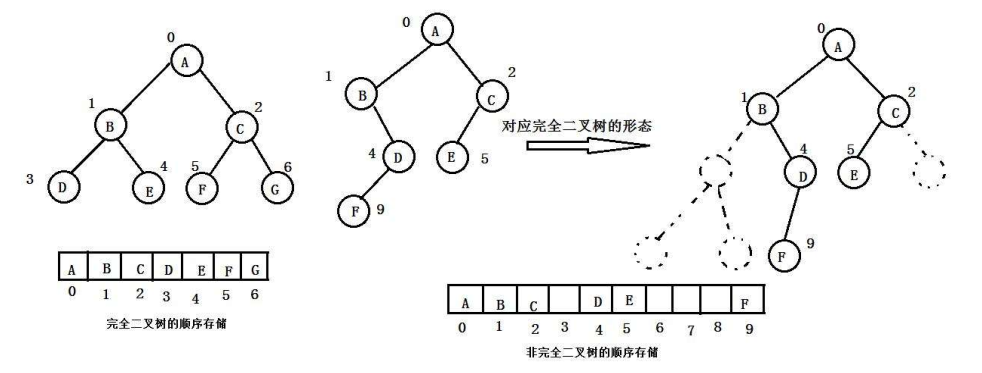2. 链式存储
二叉树的链式存储结构是指，用链表来表示一棵二叉树，即用链来指示元素的逻辑关系。 通常的方法是链表中每个结点由三个域组成，数据域和左右指针域，左右指针分别用来给出该结点左孩子和右孩子所在的链结点的存储地址 。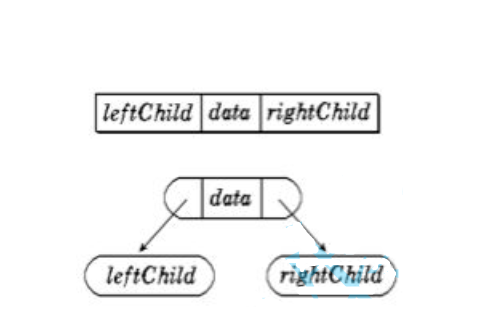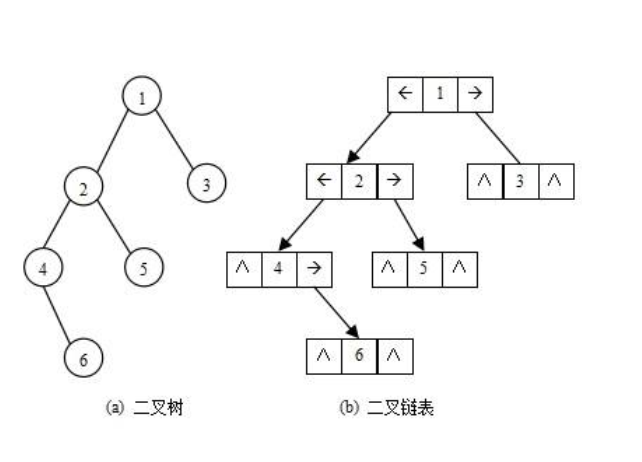``````typedef int BTDataType;
// 二叉链
struct BinaryTreeNode
{
struct BinTreeNode* _pLeft; // 指向当前节点左孩子
struct BinTreeNode* _pRight; // 指向当前节点右孩子
BTDataType _data; // 当前节点值域
}
``````

# 3.二叉树的顺序结构及实现

## 3.1二叉树的顺序结构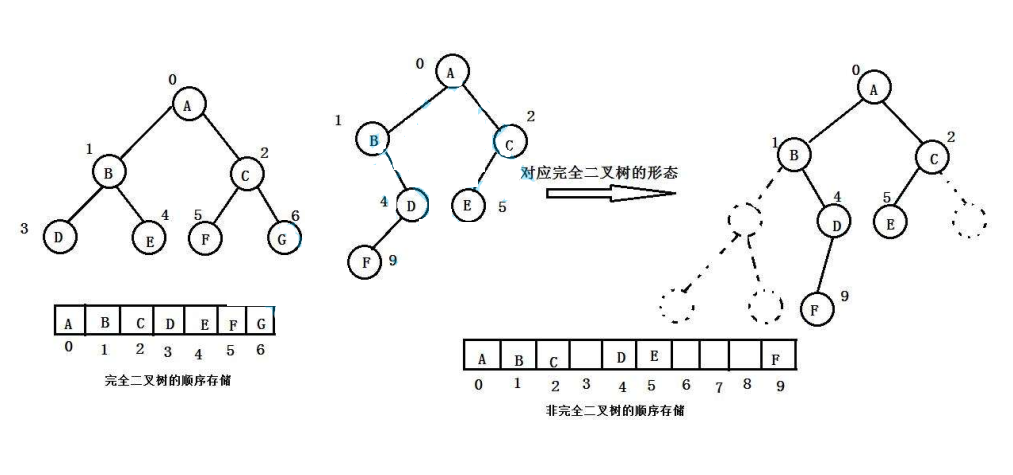## 3.2堆的概念及结构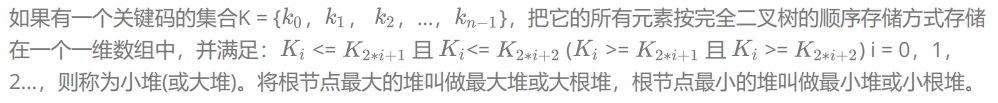1、堆中某个节点的值总是不大于或不小于其父节点的值；
2、堆总是一棵完全二叉树。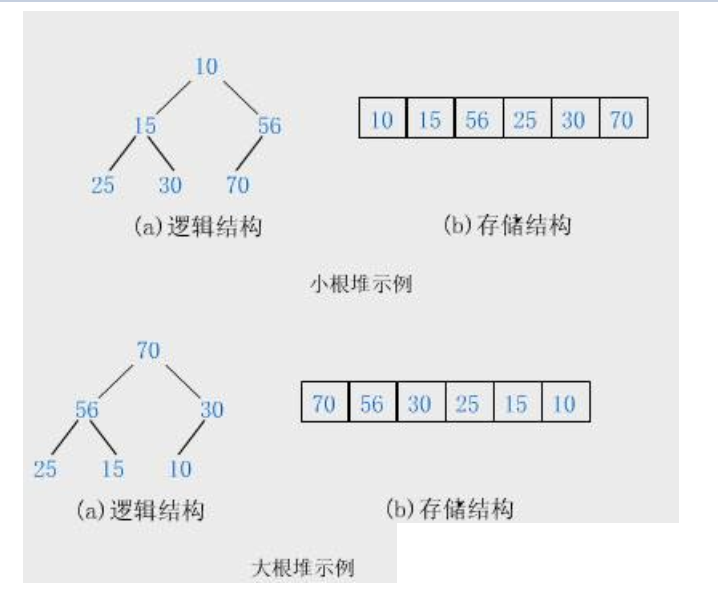## 3.3堆的实现

### 3.3.1堆向下调整算法

int array[] = {27,15,19,18,28,34,65,49,25,37};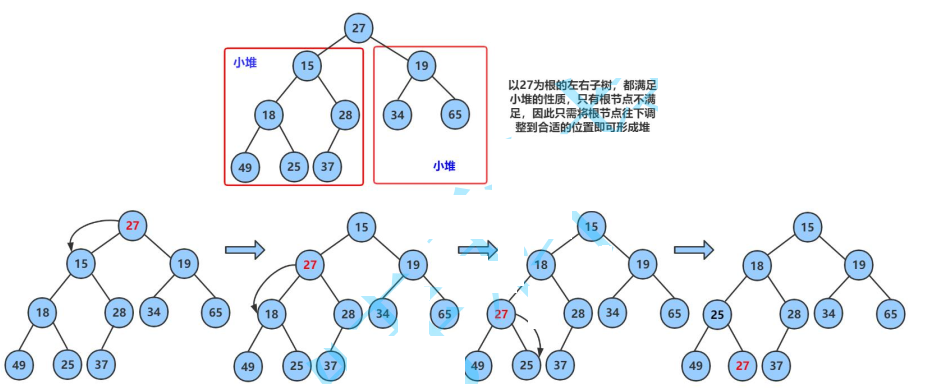### 3.3.2堆的创建

int a[] = {1,5,3,8,7,6};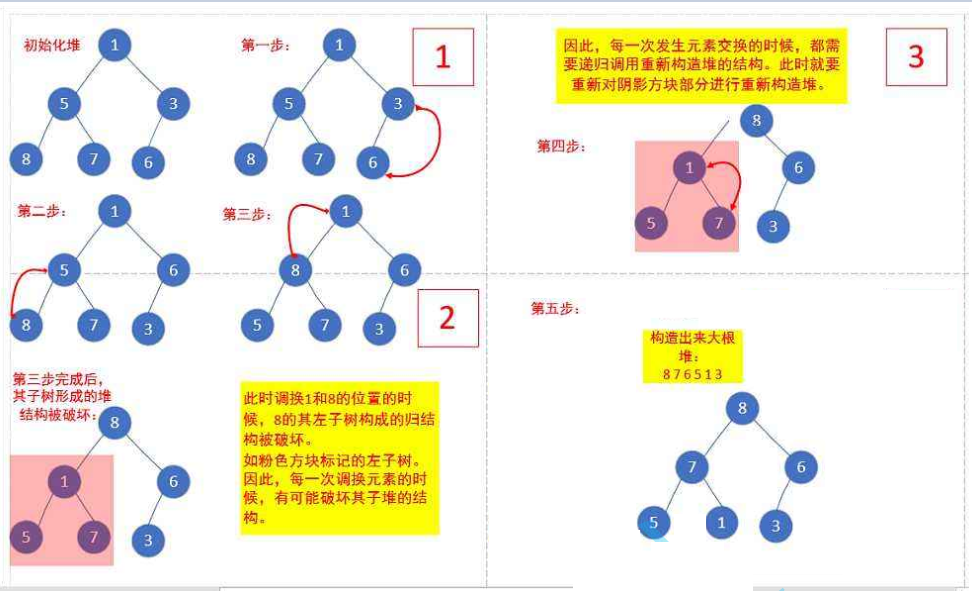### 3.3.3建堆时间复杂度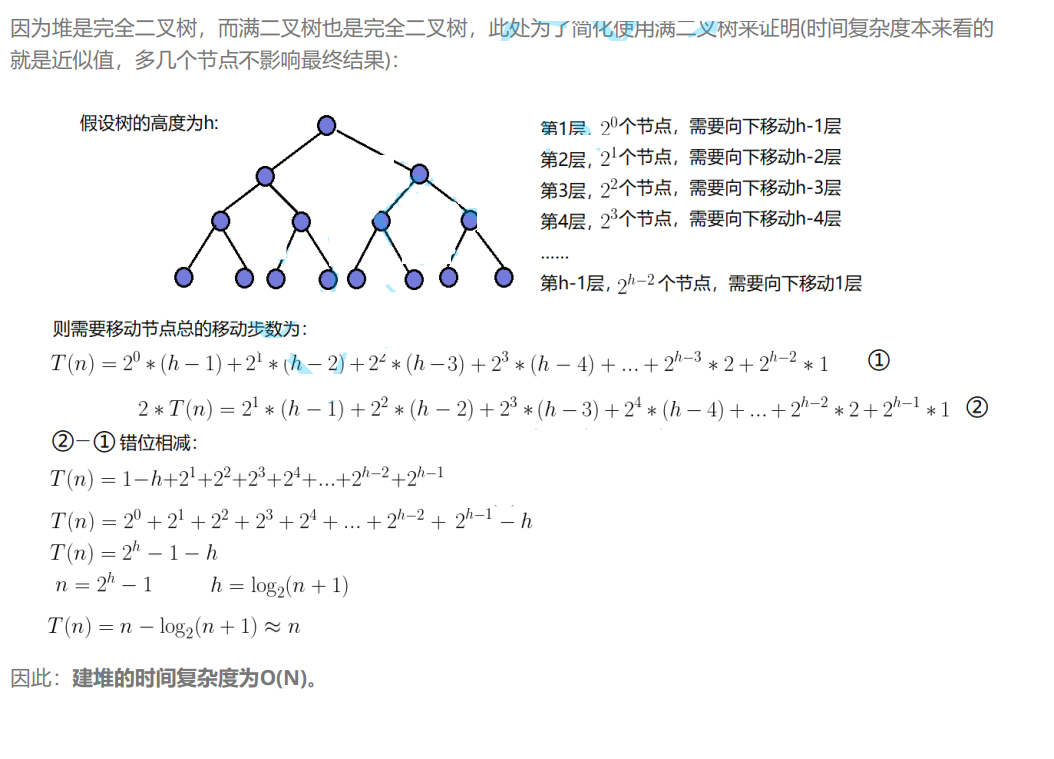### 3.3.4堆的插入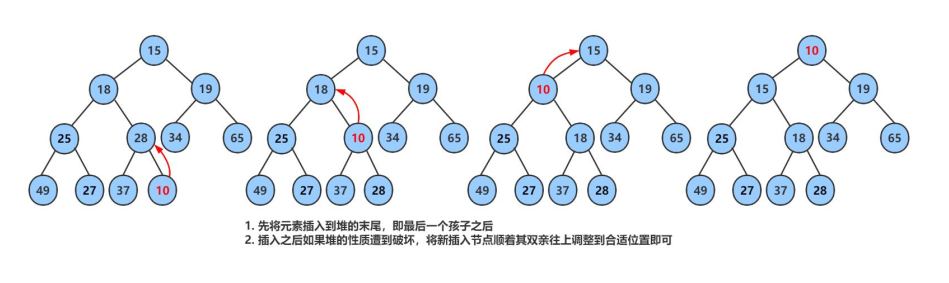### 3.3.5堆的删除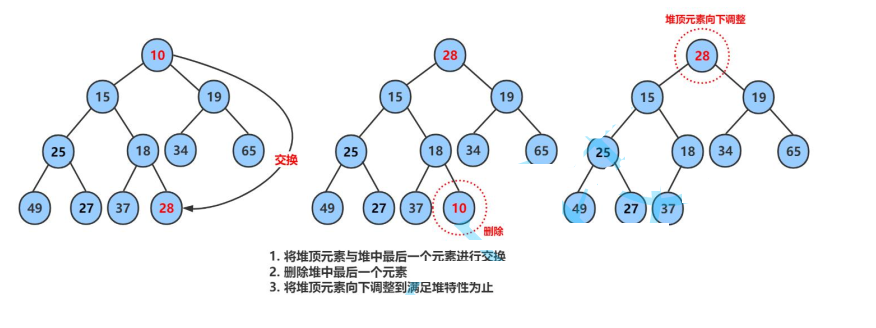### 3.3.6堆的代码实现

``````typedef int HPDataType;
typedef struct Heap
{
HPDataType* a;
int size;
int capacity;
}HP;

//交换函数
void Swap(int* px, int* py);
//向下调整算法
void AdjustDown(int* a, int n, int parent);
//向上调整算法
//堆初始化
//void HeapInit(HP* php);
void HeapInit(HP* php,HPDataType* a,int n);
//堆销毁
void HeapDestroy(HP* php);
//堆的插入，插入x，保持它继续是堆
void HeapPush(HP* php, HPDataType x);
//堆的删除，删除堆顶数据，删除后保持它继续是堆
void HeapPop(HP* php);
//获得堆顶数据，也就是最值
HPDataType HeapTop(HP* php);
//堆的判空
bool HeapEmpty(HP* php);
//求堆有多少的元素
int HeapSize(HP* php);
//堆的打印
void HeapPrint(HP* php);
``````

``````void Swap(int* px, int* py)
{
int tmp = *px;
*px = *py;
*py = tmp;
}
``````

``````//条件:左右子树都是小堆/大堆
//示例:小堆
void AdjustDown(int* a, int n, int parent)
{
int child = parent * 2 + 1;
while (child<n)
{
//选出左右孩子中小的那个
if (child + 1 < n && a[child + 1] > a[child])//判断是否越界
{
++child;
}
//1.如果小的孩子小于父亲，则交换，继续往下调整。
//2.如果小的孩子大于父亲，则结束。
if (a[child]>a[parent])
{
Swap(&a[child], &a[parent]);
parent = child;
child = parent * 2 + 1;
}
else
{
break;
}
}
}
``````

``````void AdjustUp(int* a,int child)
{
int parent = (child - 1) / 2;
while (child>0)
{
if (a[child] > a[parent])
{
Swap(&a[child], &a[parent]);
child = parent;
parent = (child - 1) / 2;
}
else
{
break;
}
}
}
``````

``````void HeapInit(HP* php, HPDataType* a, int n)
{
assert(php);
php->a = (HPDataType*)malloc(sizeof(HPDataType)*n);
if (php->a == NULL)
{
printf("malloc fail\n");
exit(-1);
}
memcpy(php->a, a, sizeof(HPDataType)*n);
//建堆
for (int i = (n - 2) / 2; i >= 0; i--)
{
}
php->size = n;
php->capacity = n;
}
``````

``````void HeapDestroy(HP* php)
{
assert(php);
free(php->a);
php->a = NULL;
php->size = php->capacity = 0;
}
``````

``````//插入x，保持它继续是堆
void HeapPush(HP* php, HPDataType x)
{
assert(php);
if (php->size == php->capacity)
{
HPDataType* tmp = (HPDataType*)realloc(php->a, php->capacity * 2 * sizeof(HPDataType));
if (php->a == NULL)
{
printf("realloc fail\n");
exit(-1);
}
php->capacity *= 2;
}
php->a[php->size] = x;
php->size++;

}
``````

``````//删除堆顶数据，删除后保持它继续是堆
void HeapPop(HP* php)
{
assert(php);
assert(!HeapEmpty(php));

Swap(&php->a, &php->a[php->size - 1]);
php->size--;

}
``````

``````//获得堆顶数据，也就是最值
HPDataType HeapTop(HP* php)
{
assert(php);
assert(!HeapEmpty(php));
return php->a;
}
``````

``````bool HeapEmpty(HP* php)
{
assert(php);
return php->size == 0;
}
``````

``````int HeapSize(HP* php)
{
assert(php);
return php->size;
}
``````

``````void HeapPrint(HP* php)
{
for (int i = 0; i < php->size; i++)
{
printf("%d ", php->a[i]);
}
printf("\n");
}
``````

## 3.4堆的应用

### 3.4.1堆排序

1. 建堆
升序：建大堆
降序：建小堆
示例：
``````//堆排序 -> 效率更高
//排升序->大堆
//排降序->小堆
void HeapSort(int* a, int n)
{
//建堆算法
for (int i = (n - 1 - 1) / 2; i >= 0; i--)
{
}
//降序
int end = n - 1;
while (end > 0)
{
Swap(&a, &a[end]);
end--;
}
}
``````
1. 利用堆删除思想来进行排序
建堆和堆删除中都用到了向下调整，因此掌握了向下调整，就可以完成堆排序。

### 3.4.2TOP-K问题

TOP-K问题：即求数据结合中前K个最大的元素或者最小的元素，一般情况下数据量都比较大。

1. 用数据集合中前K个元素来建堆
(1)前k个最大的元素，则建小堆
(2)前k个最小的元素，则建大堆
2. 用剩余的N-K个元素依次与堆顶元素来比较，不满足则替换堆顶元素
将剩余N-K个元素依次与堆顶元素比完之后，堆中剩余的K个元素就是所求的前K个最小或者最大的元素。
``````void PrintTopK(int* a, int n, int k)
{
HP hp;
HeapInit(&hp, a, k);

for (int i = k; i < n; ++i)
{
if (a[i] > HeapTop(&hp))
{
HeapPop(&hp);
HeapPush(&hp, a[i]);
}
}

HeapPrint(&hp);

HeapDestroy(&hp);
}

void TestTopk()
{
int n = 100000;
int* a = (int*)malloc(sizeof(int)*n);
srand(time(0));
for (size_t i = 0; i < n; ++i)
{
a[i] = rand() % 1000000;
}
a = 1000000 + 1;
a = 1000000 + 2;
a = 1000000 + 3;
a = 1000000 + 4;
a = 1000000 + 5;
a = 1000000 + 6;
a = 1000000 + 7;
a = 1000000 + 8;
a = 1000000 + 9;
a = 1000000 + 10;

PrintTopK(a, n, 10);
}
``````

# 4.二叉树链式结构的实现

## 4.1前置说明

``````typedef char BTDataType;
typedef struct BinaryTreeNode
{
BTDataType _data;
struct BinaryTreeNode* _left;
struct BinaryTreeNode* _right;
}BTNode;

{
BTNode* node = malloc(sizeof(BTNode));
node->_data = x;
node->_left = NULL;
node->_right = NULL;

return node;
}

BTNode* CreatBinaryTree()
{

node1->_left = node2;
node1->_right = node3;
node2->_left = node4;
node3->_left = node5;
node3->_right = node6;
return node1;
}
``````

## 4.2二叉树的遍历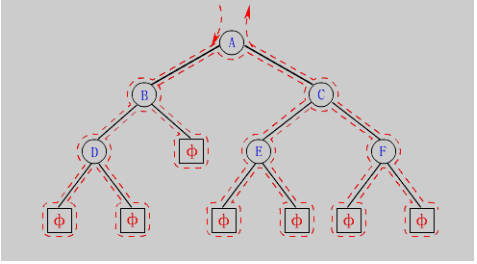1. 前序遍历(Preorder Traversal 亦称先序遍历)——访问根结点的操作发生在遍历其左右子树之前。
2. 中序遍历(Inorder Traversal)——访问根结点的操作发生在遍历其左右子树之中（间）。
3. 后序遍历(Postorder Traversal)——访问根结点的操作发生在遍历其左右子树之后。
由于被访问的结点必是某子树的根，所以N(Node）、L(Left subtree）和R(Right subtree）又可解释为根、根的左子树和根的右子树。NLR、LNR和LRN分别又称为先根遍历、中根遍历和后根遍历。
``````//前序遍历
void PreOrder(BTNode* root) {
if (root == NULL) {
printf("NULL ");
return;
}

printf("%c ", root->_data);
PreOrder(root->_left);
PreOrder(root->_right);
}

//中序遍历
void PreOrder(BTNode* root) {
if (root == NULL) {
printf("NULL ");
return;
}
PreOrder(root->_left);
printf("%c ", root->_data);
PreOrder(root->_right);
}

//后序遍历
void PreOrder(BTNode* root) {
if (root == NULL) {
printf("NULL ");
return;
}

PreOrder(root->_left);
PreOrder(root->_right);
printf("%c ", root->_data);
}
``````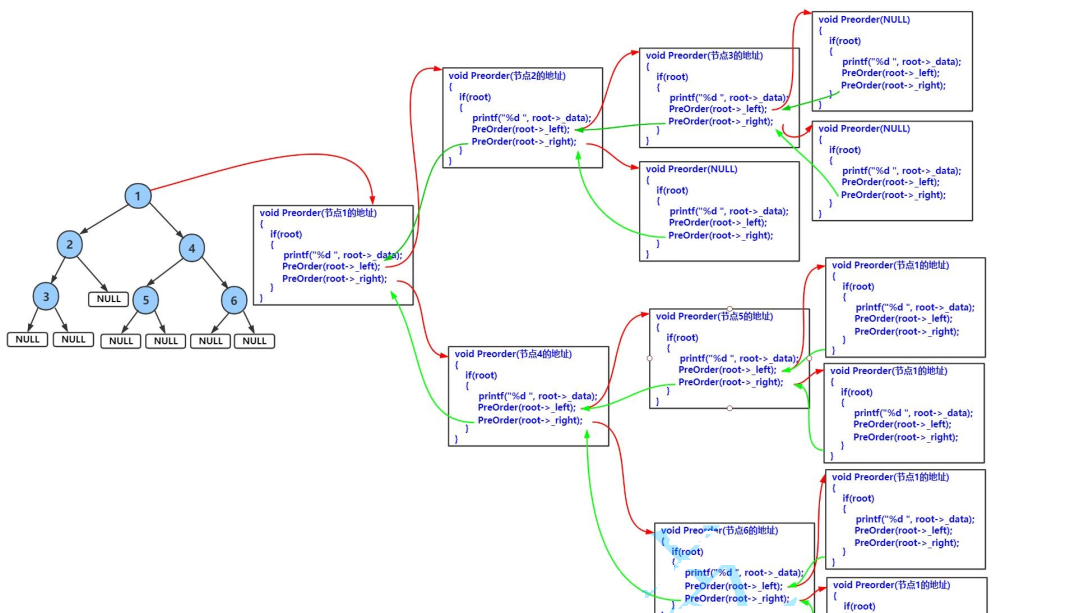``````//层序遍历
void BinaryTreeLevelOrder(BTNode* root)
{
Queue q;
QueueInit(&q);
if (root)
{
QueuePush(&q, root);
}
while (!QueueEmpty(&q))
{
BTNode* front = QueueFront(&q);
QueuePop(&q);
printf("%c ", front->data);

if (front->left)
{
QueuePush(&q, front->left);
}
if (front->right)
{
QueuePush(&q, front->right);
}
}
printf("\n");
QueueDestory(&q);
}
``````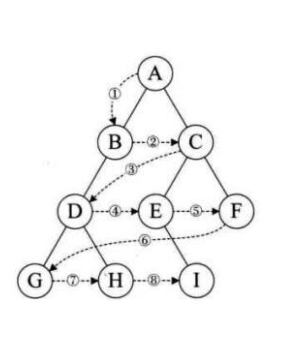## 4.3二叉树的实现

``````//二叉树节点个数
//1.遍历 -- 全局变量
//int size = 0;
//void BinaryTreeSize(BTNode* root)
//{
//	if (root == NULL)
//	{
//		return;
//	}
//	else
//	{
//		size++;
//	}
//	BinaryTreeSize(root->left);
//	BinaryTreeSize(root->right);
//
//}

//1.遍历 -- 局部变量，传地址
//void BinaryTreeSize(BTNode* root,int* psize)
//{
//	if (root == NULL)
//	{
//		return;
//	}
//	else
//	{
//		(*psize)++;
//	}
//	BinaryTreeSize(root->left,psize);
//	BinaryTreeSize(root->right,psize);
//
//}

//1.遍历 -- 递归(分而治之)
int BinaryTreeSize(BTNode* root)
{
return root == NULL ? 0 : 1 + BinaryTreeSize(root->left) + BinaryTreeSize(root->right);

}

``````

``````
//二叉树叶子节点个数
int BinaryTreeLeafSize(BTNode* root)
{
if (root == NULL)
return 0;
if (root->left == NULL && root->right == NULL)
return 1;
return BinaryTreeLeafSize(root->left) + BinaryTreeLeafSize(root->right);
}
``````

``````//二叉树第k层节点个数
//核心思路：求当前树的第k层 = 左子树的第k-1层 + 右子树的第k-1层。
int BinaryTreeLevelKSize(BTNode* root, int k)
{
if (root == NULL)
return 0;
if (k == 1)
return 1;
return BinaryTreeLevelKSize(root->left, k - 1) + BinaryTreeLevelKSize(root->right, k - 1);
}
``````

``````//二叉树深度/高度
int BinaryTreeDepth(BTNode* root)
{
if (root == NULL)
return 0;
int leftDepth = BinaryTreeDepth(root->left);
int rightDepth = BinaryTreeDepth(root->right);
return leftDepth > rightDepth ? leftDepth + 1 : rightDepth + 1;
}
``````

``````// 二叉树查找值为x的节点
//先判断是不是当前节点，是就返回；不是先去左树找，找到了就返回；左树没找到，再去右树找。
BTNode* BinaryTreeFind(BTNode* root, BTDataType x)
{
if (root == NULL)
{
return NULL;
}
if (root->data == x)
{
return root;
}
BTNode* retleft = BinaryTreeFind(root->left, x);
if (retleft)
{
return retleft;
}
BTNode* retright = BinaryTreeFind(root->right, x);
if (retright)
{
return retright;
}
return NULL;
}
``````

``````// 二叉树销毁
void BinaryTreeDestory(BTNode* root)
{
if (root == NULL)
{
return;
}
BinaryTreeDestory(root->left);
BinaryTreeDestory(root->right);
free(root);
}
``````

``````//判断二叉树是否是完全二叉树
//核心思路：层序遍历，把空也入队列；完全二叉树，非空是连续；不是完全二叉树，非空不是连续。
bool BinaryTreeComplete(BTNode* root)
{
Queue q;
QueueInit(&q);
if (root)
{
QueuePush(&q, root);
}
while (!QueueEmpty(&q))
{
BTNode* front = QueueFront(&q);
QueuePop(&q);
if (front == NULL)
{
break;
}
QueuePush(&q, front->left);
QueuePush(&q, front->right);
}
//出到空，以后，队列中全是空，就是完全二叉树；
//还有非空，就不是完全二叉树。
while (!QueueEmpty(&q))
{
BTNode* front = QueueFront(&q);
QueuePop(&q);
if (front)
{
QueueDestory(&q);
return false;
}

}
QueueDestory(&q);
return true;
}
``````

<< 上一篇 下一篇 >>

### 评论（0） 赞助本站◎欢迎参与讨论，请在这里发表您的看法、交流您的观点。